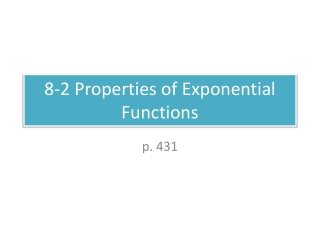DownloadDownload Presentation8-2 Properties of Exponential Functions

# 8-2 Properties of Exponential Functions

Download Presentation## 8-2 Properties of Exponential Functions

- - - - - - - - - - - - - - - - - - - - - - - - - - - E N D - - - - - - - - - - - - - - - - - - - - - - - - - - -
##### Presentation Transcript

1. Do Now Does the function represent growth or decay? What is the percent of increase or decrease? GROWTH a = 5 b = 3/2 GREATHER THAN 1!

2. Example 1Graph. Make a table of values for ANY exponential function!

3. e • e is a real number constant that is useful for describing exponential growth or decay. CONTINUOUSLY COMPOUNDED INTEREST FORMULA: A = Pert Constant Time in years Annual interest rate Amount in the account Principal amount

4. Example 2 Suppose you invest \$1300 at an annual interest rate of 4.3% compounded continuously. Find the amount in the account after 3 years. A = ? P = 1300 r = .043 t = 3 A = (1300)e.043(3) A = Pert = \$1479

5. Example 3 Sodium-24 has a half-life of 15 hours. How much sodium-24 will you have after 60 hours if your original sample is 64mg? How many half-lives in a 60 hour period? Let y = the amount of sodium-24 Let x = the number of hours elapsed AWAYS ½!

6. Homework • p. 426 #1-33 every other odd • p. 434 #1-25 every other odd